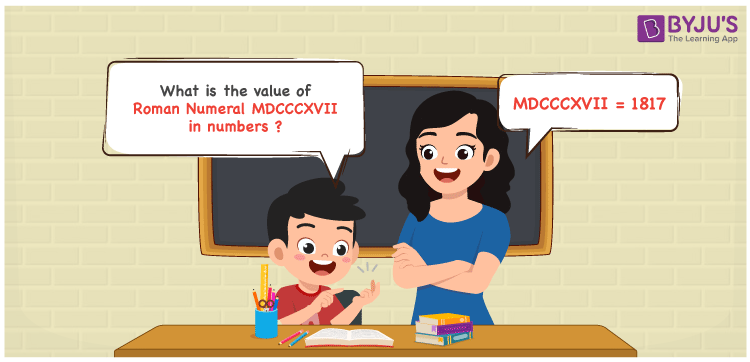Checkout JEE MAINS 2022 Question Paper Analysis : Checkout JEE MAINS 2022 Question Paper Analysis :

# MDCCCXVII Roman Numerals

MDCCCXVII Roman Numerals is 1817. This article gives the steps used to convert the Roman numerals MDCCCXVII in numeric form according to the grasping abilities of students. The roman numerals conversion can be used to get your queries clarified in a short time. Therefore, the roman numerals MDCCCXVII is written as 1817 in numbers.

 Number Roman Numeral 1817 MDCCCXVII## How to Write MDCCCXVII Roman Numerals in Numbers?

This section gives students a clear knowledge of the conversion used to write MDCCCXVII to numbers.

MDCCCXVII = M + D + C + C + C + X + V + I + I

MDCCCXVII = 1000 + 500 + 100 + 100 + 100 + 10 + 5 + 1 + 1

MDCCCXVII = 1817

## Video Lesson on Roman Numerals## Frequently Asked Questions on MDCCCXVII Roman Numerals

### Why is MDCCCXVII the roman numerals of 1817?

We know that

M is the roman numerals of 1000

DCCC is the roman numerals of 800

X is the roman numerals of 10

VII is the roman numerals of 7

So MDCCCXVII is the roman numeral of 1817.

### Determine 1800 + 17.

We know that

1800 + 17 = 1817

Hence, 1800 + 17 is 1817 which is written as MDCCCXVII.

### Find the remainder if MDCCCXVII is divided by X.

We know that

MDCCCXVII = 1817

X = 10

The remainder if MDCCCXVII is divided by X is VII.